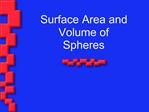DownloadDownload PresentationSurface Area and Volume of Spheres

# Surface Area and Volume of Spheres

Télécharger la présentation## Surface Area and Volume of Spheres

- - - - - - - - - - - - - - - - - - - - - - - - - - - E N D - - - - - - - - - - - - - - - - - - - - - - - - - - -
##### Presentation Transcript

1. Surface Area and Volume of Spheres

2. Surface Area Sphere

3. Finding the Surface Area of a Sphere The point is called the center of the sphere. A radius of a sphere is a segment from the center to a point on the sphere. A chord of a sphere is a segment whose endpoints are on the sphere.

4. Surface Area of a Sphere The surface area of a sphere with radius r is S = 4?r2.

5. Your Turn!!! Find the surface area. When the radius doubles, does the surface area double?

6. SA = 4?r2 = (4)(3.14)22 = 50.24 in.2 SA = 4?r2 = (4)(3.14)42 = 200.16 in.2

7. Volume of a Sphere The volume of a sphere with radius r is V = 4?r3.

8. Volume of a Sphere Find the volume of a sphere with a radius of 10m.

9. Surface Area and Volume of Spheres